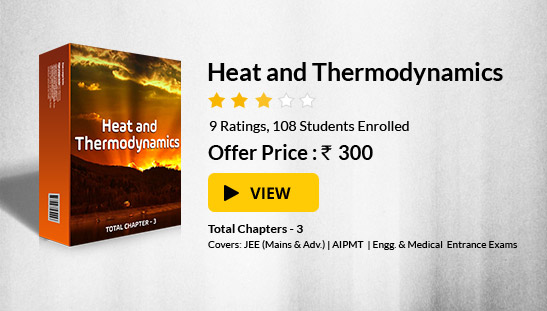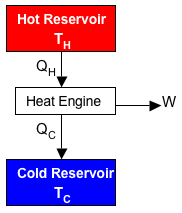Click to Chat

1800-1023-196

+91-120-4616500

CART 0

• 0

MY CART (5)

Use Coupon: CART20 and get 20% off on all online Study Material

ITEM
DETAILS
MRP
DISCOUNT
FINAL PRICE
Total Price: Rs.

There are no items in this cart.
Continue Shopping• Complete Physics Course - Class 11
• OFFERED PRICE: Rs. 2,968
• View Details

```Second Law of Thermodynamics

Table of Content

Entropy

Co-Efficient of Performance (K)

Related Resources

EntropyThe physical quantity entropy is a measureable property and describes the ability of the system to do work. Entropy of a substance is, therefore, said to be a measure of the degree of disorder prevailing among its molecules, just as the temperature is a measure of the degree of hotness of a substance. At the absolute zero of temperature the thermal motion completely disappears so that the disorder and hence the entropy tends to zero and the molecules of a substance are in perfect order, i.e., well arranged. Higher the entropy, higher is the disorder. The entropy of any isolated system increases and approaches, more or less rapidly, to the inert state of maximum entropy.

Mathematically,

dS = dQ/T

In terms of entropy the second law of thermodynamics may be stated as follows:-

The entropy of an isolated system is fully conserved in every reversible process, i.e. for every reversible process the sum of all changes in entropy taking place in an isolated system is zero. If the process is not reversible one, then the sum of all changes in entropy taking place in an isolated system is greater than zero. In general we can say that in every process taking place in an isolated system the entropy of the system either increases or remains constant.

Furthermore, if an isolated system is in such a state that its entropy is a maximum, any change from that state would necessarily involve a decrease in entropy and hence will not happen. Therefore, the necessary condition for an equilibrium of an isolated system is that its entropy shall be maximum.

It is to be noted that the above statements are applicable to isolated system only. The more usual forms of the second law of thermodynamics are as follows.

(a) It is impossible to construct a device which, operating in a cycle has the sole effect of extracting heat from a single reservoir and performing an equivalent amount of work. (Kelvin-Planck Statement)

Efficiency of an engine is 1 only if the sink is at absolute zero. Since absolute zero is only a theoretical concept, η (ε) = 1 for an engine is not possible.

Since, η = 1 – Q2/Q1

Therefore, ‘Q2’ can never be equal to ‘Q1’, i.e., some amount of heat has to be rejected to the sink. Hence whole of the extracted heat cannot be converted into work.

(b) Heat cannot flow from a cold body to a hot body without the performance of work by some external agency. (Clausius Statement)

Efficiency ‘η’ of the engine is, η = 1 – Q2/Q1

For the transference of heat from a cold body to a hot body , the temperature T2 of the sink has to be greater than T1 of the source, i.e., T2>T1. In that ‘η’  becomes negative which is meaningless concept.

Most of these limitations of the 1st law of thermodynamics have been taken care off in the second law. The second law of thermodynamics helps us in estimating whether the reaction is actually viable or not and it also tells the direction of heat. The second law also clarifies that it is not possible to convert energy completely into work.Co-efficient of Performance (K)

Co-efficient of performance of a refrigerator is defined as the amount of heat removed per unit work done on the machine.

K = Heat removed / Work done

= Q2/W

Since, W = Q1 - Q2

So, K = Q2/Q1 - Q2

= 1 / [(Q1/Q2) – 1]

= Q2/Q1 - Q2

= QL / QH – QL

Or, K = 1 / [(T1/T2) – 1]

=  T2/T1 - T2 = TL / TH – TL

Problem 1:- A mixture of 1.78 kg of water and 2.62 g of ice at 0°C is, in a reversible process, brought to a final equilibrium state where the water/ice ratio, by mass, is 1:1 at 0°C. (a) Calculate the entropy change of the system during this process. (b) The system is then returned to the first equilibrium state, but in an irreversible way (by using Bunsen burner, for instance). Calculate the entropy change of the system during this process. (c) Show that your answer is consistent with the second law of thermodynamics.

Concept:-

The entropy change ΔS for a reversible isothermal process is defined as,

ΔS = Q/T

= -mL/T

Here m is the mass, L is the latent heat and T is the temperature.

Solution:-

(a) Mass of water = 1.78 kg

Mass of ice = 262 g

So the total mass of ice and water mixture will be,

Mass of ice-water mixture = (Mass of water) + (Mass of ice)

= (1.78 kg) + (262 g)

= (1.78 kg) + (262 g×10-3 kg/1 g)

= 1.78 kg + 0.262 kg

= 2.04 kg

If eventually the ice and water have the same mass, then the final state will have 1.02 kg (2.04 kg/2) of each.

Thus the mass of the water that changed into ice m will be the difference of mass of water mw  and mass of final state ms.

So, m = mw - ms

To obtain mass of water that changed into ice m, substitute 1.78 kg for mass of water mw and 1.02 kg for mass of final state ms in the equation m = mw - ms,

m = mw - ms

= 1.78 kg – 1.02 kg

= 0.76 kg

The change of water at 0° C to ice at 0° C  is isothermal.

To obtain the change in entropy ΔS of the system during this process, substitute 0.76 kg for mass m, 333×103 J/kg for heat of fusion of water L and 273 K for T in the equation ΔS = -mL/T,

ΔS = -mL/T

= -(0.76 kg) (333×103 J/kg )/(273 K)

= -927 J/K

From the above observation we conclude that, the change in entropy ΔS of the system during this process will be -927 J/K.

(b)

Now the system is returned to the first equilibrium state, but in an irreversible way. Thus the change in entropy ΔS of the system during this process is equal to the negative of previous case.

So, ΔS = -(- 927 J/K)

= 927 J/K

From the above observation we conclude that, the change in entropy ΔS of the system would be 927 J/K.

(c)  In accordance to second law of thermodynamics, entropy change ΔS is always      zero.

The total change in entropy will be,

ΔS = (-927 J/K) + (927 J/K)

= 0

From the above observation we conclude that, our answer is consistent with the second law of thermodynamics.

Problem 2:-

Engine A, compared to engine B, produces, per cycle, five times the work but receives three times the heat input and exhausts out twice the heat. Determine the efficiency of each engine.

Concept:-

Work done in an engine is equal to the difference of input heat and output heat.

Efficiency εA of engine A is equal to,

εA = WA/ Qi,A

Here, WA is the work done by engine A and Qi,A is the input heat of engine A.

Efficiency εB of engine B is equal to,

εB = WB/ Qi,B

Here, WB is the work done by engine B and Qi,B is the input heat of engine B.

Solution:-

Let work done by the engine B is WB. Since the engine A produces, per cycle, five times the work of B, so the work done WA by the engine A will be,

WA = 5 WB

Let heat input of engine B is Qi,B. Since engine A receives three times heat input of B, so the heat input Qi,A of engine A will be,

Qi,A = 3 Qi,B

Let heat output of engine B is Qo,B. Since engine A exhausts two times heat output of B, so the heat input Qo,A of engine A will be,

Qo,A = 2 Qo,B

Work done in an engine is equal to the difference of input heat and output heat.

So, WA = Qi,A - Qo,A

and

WB = Qi,B - Qo,B

Qo,B = Qi,B -WB

Substitute 5 WB for WA, 3 Qi,B for Qi,A and 2 Qo,B for Qo,A in the equation WA = Qi,A - Qo,A, we get,

WA = Qi,A - Qo,A

5 WB = 3 Qi,B - 2 Qo,B

= 3 Qi,B – 2(Qi,B -WB)  (Since, Qo,B = Qi,B -WB)

3 WB  = Qi,B

So, efficiency of engine B, εB will be,

εB = WB/ Qi,B

= 1/3

And

efficiency of engine A, εA will be,

εA = WA/ Qi,A

= 5 WB/3 Qi,B       (Since, WA = 5 WB and Qi,A = 3 Qi,B)

= (5/3) (1/3)       (Since,WB/Qi,B = 1/3)

= 5/9

From the above observation we conclude that, the efficiency of engine A will be 5/9 and engine B will be 1/3.

Problem 3:-

To make some ice, a freezer extracts 185 kJ of heat at -12.0°C. The freezer has a coefficient of performance of 5.70. The room temperature is 26.0°C. (a) How much heat is delivered to the rom? (b) How much work is required to run the freezer?

Concept:-

A freezer would like to extract as much heat QL as possible from the low-temperature reservoir (“what you want”) for the least amount of work W (“what you pay for”). So the efficiency of a freezer is defined as,

K = (what you want)/(what you pay for)

= QL/W

and this is called coefficient of performance. The larger the value of K, the more efficient is the refrigerator.

Thus, W = QL/K

The first law of thermodynamics, applied to the working substance of the freezer, gives

W = QH – QL

Here QH is the exhausted heat.

Thus exhausted heat will be,

QH = W + QL

Solution:-

(a) To obtain the heat that is delivered to the room, first we have to find out the required work W to run the freezer.

To obtain the required work W to run the freezer, substitute 185 kJ for extracted heat QL and 5.70 for the coefficient of performance K in the equation W = QL/K,

W = QL/K

= 185 kJ/5.70

= 32.5 kJ

To obtain the heat that is delivered to the room, substitute 32.5 kJ for work W which is required to run the freezer and 185 kJ for extracted heat QL and in the equation QH = W + QL,

QH = W + QL

=32.5 kJ + 185 kJ

= 217.5 kJ

Rounding off to three significant figures, the heat delivered to the room would be 218 kJ.

(b) To obtain the required work W to run the freezer, substitute 185 kJ for extracted heat QL and 5.70 for the coefficient of performance K in the equation W = QL/K,

W = QL/K

= 185 kJ/5.70

= 32.5 kJ

Therefore the required work W to run the freezer would be 32.5 kJ.

Related Resources

You might like to refer some of the related resources listed below:

Look into the Sample Papers of Previous Years to get a hint of the kinds of questions asked in the exam.

To read more, Buy study materials of Thermodynamics comprising study notes, revision notes, video lectures, previous year solved questions etc. Also browse for more study materials on Chemistry here.
```### Course Features

• 101 Video Lectures
• Revision Notes
• Previous Year Papers
• Mind Map
• Study Planner
• NCERT Solutions
• Discussion Forum
• Test paper with Video Solution